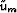Next: REFERENCES Up: A surface-related multiple prediction Previous: 2- and 3-D earth

# Limitations of the multiple prediction using real data

In the real life, the source is not impulsive. In addition, multiples are computed directly from the data and not from the primary wavefield. In that, a model of the multiples is obtained by convolving the recorded data with primaries and multiples as opposed to the primaries only. Hence, the relative amplitude of first order multiples with respect to higher order multiples is not preserved. To illustrate this last point, consider the surface-related multiple modeling equation Verschuur et al. (1992)

 ur = u0 - W-1 um, (151)

where ur is the recorded wavefield at the surface with primaries and surface-related multiples, W the source wavelet, and um the surface-related multiple wavefield given by(152) (153)
whererepresents the nonstationary convolution and ui the i-th order multiples. If we use equation (), replacing u0 by ur, we obtain for the approximated multiple field(154)
Comparing equation () and equation (), we notice that higher order multiples in equation () are multiplied by a coefficient that is difficult to correct for. Therefore higher order multiples have the correct kinematics, but the wrong amplitudes Hugonnet (2002); Wang and Levin (1994). Hence, the modeling scheme that consists in convolving the shot gathers only once explicitely overpredict high-order multiples (amplitude wise) but models them with the correct pattern. As shown by Berkhout and Verschuur (1997) and Verschuur and Berkhout (1997), this single convolution can be interpreted as a first iteration of the recursive formulation of SRME.Next: REFERENCES Up: A surface-related multiple prediction Previous: 2- and 3-D earth
Stanford Exploration Project
5/5/2005